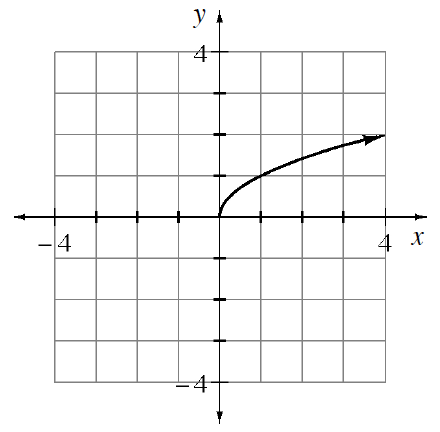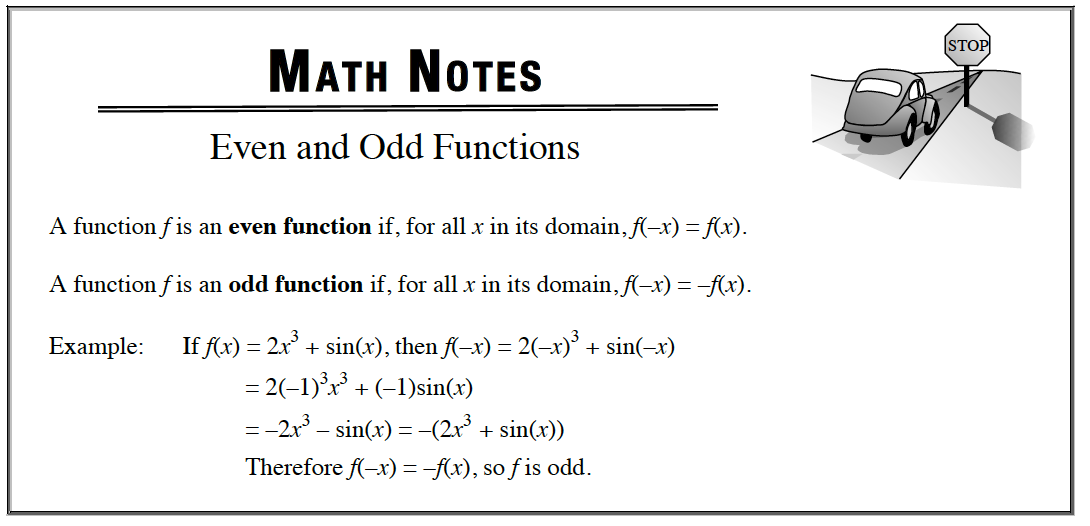### Home > APCALC > Chapter 1 > Lesson 1.2.2 > Problem1-43

1-43.

Copy the graph at right. Then complete it so it will have each type of symmetry described below. 1-43 HW eTool (Desmos). Homework Help ✎

1. Reflection symmetry across the $y$-axis.

2. Reflection symmetry across the $x$-axis.

3. Point symmetry about the origin. (This means a $180º$ rotation about the origin leaves the graph unchanged.)

4. Recall the definitions of even and odd functions. For each part above, state if the graph is even, odd, or neither.Use the eTool below to view the graphs.
Click the link at right for the full version of the eTool: Calc 1-43 HW eTool